# Koch Island (Fractals)

$\color{#D61F06}{\text{Fractals}}$ can be defined as "objects or figures which on magnification shows the original object or figure"

The nature has many fractals such as a fern leaf, path of a river etc.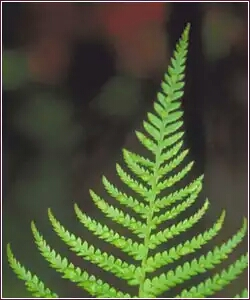A fern leaf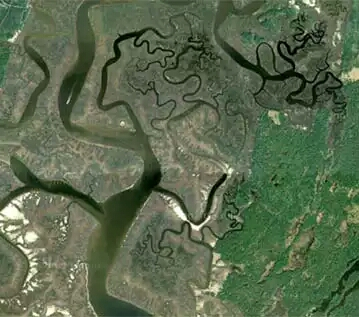A river's path

Geometrical fractals includes a straight line, today's talk $\color{#20A900}{\text{Koch Island}}$ and much more!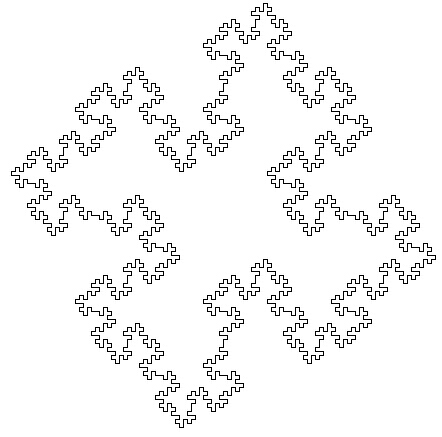Koch Island

The Koch Island is drawn by "copies of copies" method.

The base of Koch Island is a square as shown: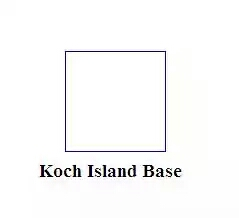Base of Koch Island

The motif of Koch Island is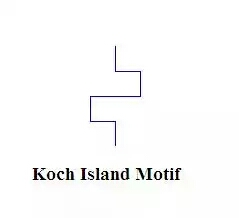Motif of Koch Island

The steps of making a Koch Island are as follows:

• Draw the base.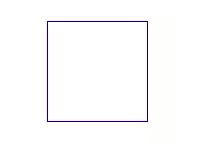Drawing a base

• Replace the sides of base by the motif (of appropriate size)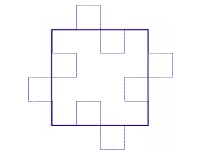Replacing sides

• Repeat the previous step again and again (By "again and again", I mean nothing less than ∞)

The figure after

• one iteration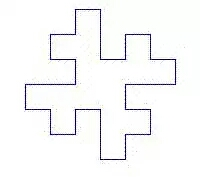After one iteration

• two iterations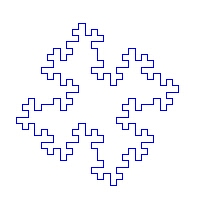After two iterations

$\color{#3D99F6}{Secret:} \color{#EC7300}{\text{Beauty} \propto \text{Number of iterations}}$

So repeat it as much as you can!!!!

One exciting fact about the Koch Island is that it can tesselate a plane.

Tesselating a plane means that a plane can be covered up by infinite Koch Islands without overlapping or leaving spaces.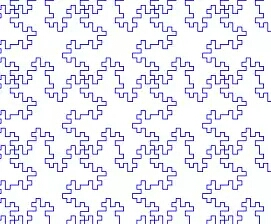Tesselations

Question 1: Can anyone guess the area and perimeter of the Koch Island after n iterations?

Question 2: What is the minimun number of colors that you could use to color in your tessellation if no two adjoining Koch Islands are allowed to be the same color (no islands can touch even at 1 point)?

Question 3: What is the self-similarity dimension of Koch Island?

Question 4: Can you offer a MSW-Logo program to draw a Koch Island?

###### Image credit:miqel.com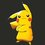Note by Pranjal Jain
5 years, 9 months ago

This discussion board is a place to discuss our Daily Challenges and the math and science related to those challenges. Explanations are more than just a solution — they should explain the steps and thinking strategies that you used to obtain the solution. Comments should further the discussion of math and science.

When posting on Brilliant:

• Use the emojis to react to an explanation, whether you're congratulating a job well done , or just really confused .
• Ask specific questions about the challenge or the steps in somebody's explanation. Well-posed questions can add a lot to the discussion, but posting "I don't understand!" doesn't help anyone.
• Try to contribute something new to the discussion, whether it is an extension, generalization or other idea related to the challenge.

MarkdownAppears as
*italics* or _italics_ italics
**bold** or __bold__ bold
- bulleted- list
• bulleted
• list
1. numbered2. list
1. numbered
2. list
Note: you must add a full line of space before and after lists for them to show up correctly
paragraph 1paragraph 2

paragraph 1

paragraph 2

[example link](https://brilliant.org)example link
> This is a quote
This is a quote
    # I indented these lines
# 4 spaces, and now they show
# up as a code block.

print "hello world"
# I indented these lines
# 4 spaces, and now they show
# up as a code block.

print "hello world"
MathAppears as
Remember to wrap math in $$ ... $$ or $ ... $ to ensure proper formatting.
2 \times 3 $2 \times 3$
2^{34} $2^{34}$
a_{i-1} $a_{i-1}$
\frac{2}{3} $\frac{2}{3}$
\sqrt{2} $\sqrt{2}$
\sum_{i=1}^3 $\sum_{i=1}^3$
\sin \theta $\sin \theta$
\boxed{123} $\boxed{123}$

Sort by:

1. Area is constant; perimeter doubles per iteration.

2. Four colours (I think).

- 5 years, 9 months ago

Both are correct! Try $3^{rd}$ and $4^{th}$ too!

- 5 years, 9 months ago

what is a self-similarity dimension?

- 5 years, 8 months ago

Self similarity Dimension is the property of a fractal which is defined as "index for characterizing fractal patterns or sets by quantifying their complexity as a ratio of the change in detail to the change in scale".

- 5 years, 8 months ago

Self similarity Dimension is the property of a fractal which is defined as "index for characterizing fractal patterns or sets by quantifying their complexity as a ratio of the change in detail to the change in scale".

- 5 years, 8 months ago

self- similarity dimension is 2?

- 5 years, 9 months ago

I dont have much idea about self-similarity dimensions. Just saw a glimpse at National Science Camp, Kolkata. Can you prove it?

- 5 years, 9 months ago

usually the self similarity dimension is given by d=log(n)/log(k) . ...... where 'd' is the dimension, 'k' is the scaling factor(actually scaled as '1/k' but taken as k) and n is the number of pieces of fractals in the figure given, resembling the original one.... It is simply like someone askin ya "hey! how many pieces of the original should i have if i scale the fractal by a factor '1/3' given its self similarity dimension is 2" ........ answer is 9 pieces. ( * agreed that fractals are the most beautiful objects ...... but theyre really too complex and scary .... have you heard of the non integral dimensions for fractals !! * )

NOTE : scaling is taken as equivalent to performing iterations.

- 5 years, 9 months ago

and yes! ..... i really loved your post, no one really speaks much about fractals. CAN YOU KEEP GOIN AND POST SOME MORE (thanks) :)

- 5 years, 9 months ago

- 5 years, 9 months ago

What would be "n" and "k" in the given fractal? Non integral dimensions?? Well no! Something new for me! Curious to know about that!

- 5 years, 9 months ago

I think it must be n=16, k=4

So $D=\dfrac{log\ 16}{log\ 4}=\boxed{2}$

- 5 years, 9 months ago

yes ure right!

- 5 years, 9 months ago

well there are many other beautiful facts .. lets take koch island as an example .... after infinite iterations it has finite area but infinite perimeter!! ..... I mean you can have different perimeter if you scale the fractal at different factors ....... to exclude this contradiction dimensions for fractals were redefined as the formula above read this for an elegant detail about non integral dimensions.

- 5 years, 9 months ago

Thanks for the info!

- 5 years, 9 months ago